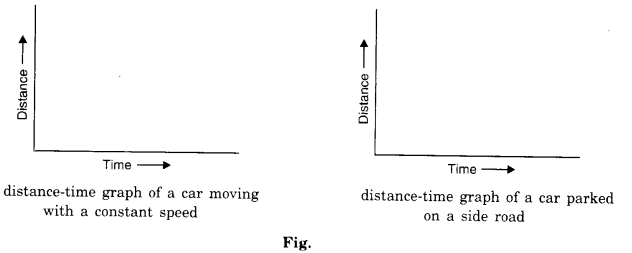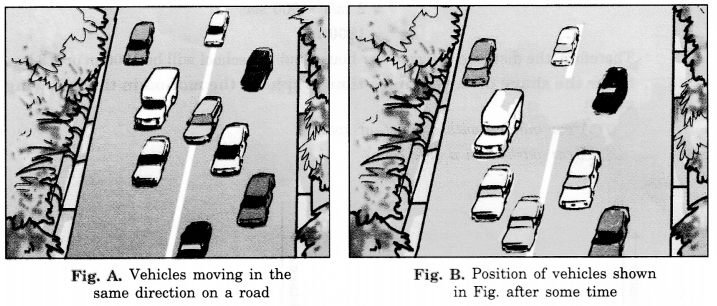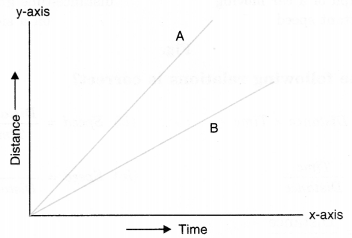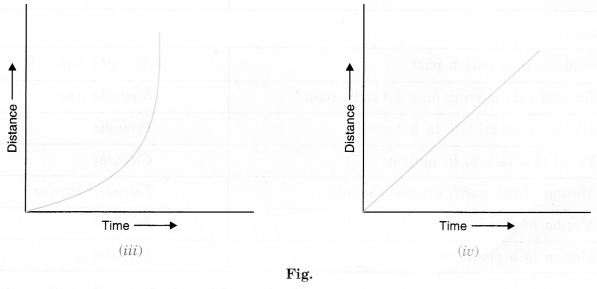NCERT Solutions for Class 7 Science Chapter 13 Motion and Time are part of NCERT Solutions for Class 7 Science. Here we have given NCERT Solutions for Class 7 Science Chapter 13 Motion and Time.

 Board CBSE Textbook NCERT Class Class 7 Subject Science Chapter Chapter 13 Chapter Name Motion and Time Number of Questions Solved 13 Category NCERT Solutions

## NCERT Solutions for Class 7 Science Chapter 13 Motion and Time

Question 1.
Classify the following as motion along a straight line, circular or oscillatory motion:

1. Motion of your hands while running.
2. Motion of a horse pulling a cart on a straight road.
3. Motion of a child in a merry-go-round.
4. Motion of a child on a see-saw.
5. Motion of the hammer of an electric bell.
6. Motion of a train on a straight bridge.

1. Oscillatory
2. Straight line
3. Circular
4. Oscillatory
5. Oscillatory
6. Straight line

Question 2.
Which of the following are not correct?
(i) The basic unit of time is second.
(ii) Every object moves at a constant speed.
(iii) Distance between two cities is measured in kilometers.
(iv) The time period of a given pendulum is not constant.
(v) The speed of a train is expressed in m/h.
(ii), (iv), (v).

Question 3.
A simple pendulum takes 32 sec. to complete 20 oscillations. What is the time period of the pendulum?
Time taken for 20 oscillations = 32 s
Time taken for 1 oscillation = $$\frac { 32 s }{ 20 }$$
Hence, Time period = 1.6 s

Question 4.
The distance between the two stations is 240 km. A train takes 4 hours to cover this distance. Calculate the speed of the train.
Given, distance = 240 km
time = 4 h
speed = $$\frac { distance }{ time }$$ = $$\frac { 240 km }{ 4 h }$$ = 60 km/h.

Question 5.
The odometer of a car reads 57321.0 km when the clock shows the time 08: 30 AM. What is the distance moved by car, if at 08: 50 AM, the odometer reading has changed to 57336.0 km? Calculate the speed of the car in km/min during this time. Express the speed in km/h also.
In this question, it is given that,
Initial reading of odometer = 57321.0 km
Final reading of odometer = 57336.0 km
Initial time = 08.30 AM
Final time = 08.50 AM
Now, total distance covered = Final reading of odometer – Initial reading of odometer
= 57336.0 km – 57321.0 km = 15.0 km
Total time taken = Final time – Initial time
= 08.50 AM – 08.30 AM
= 20 min
We know that,
speed = $$\frac { Distance\quad covered }{ Time\quad taken } =\frac { 15Km }{ 20min } =0.75Km/min$$
Speed in km/h = 0.75 x 60 (1 hr = 60 min) = 45 km/hr
Therefore, the distance moved by the car =15 km
and the speed of the car = 0.75 km/min and 45 km/h.

Question 6.
Salma takes 15 minutes from her house to reach her school on a bicycle. If the bicycle has a speed of 2 m/s, calculate the distance between her house and the school.
Given, Speed = 2 m/s
Time =15 min = 15 x 60 s = 900 s
distance = speed x time = 2 m/s x 900 s = 1800 m
$$\frac { 1800 m }{ 1000 }$$ km = 1.8 km

Question 7.
Show the shape of the distance-time graph for the motion in the following cases:
(i) A car moving at a constant speed.
(ii) A car parked on a side road.Question 8.
Which of the following relations is correct?
(i) Speed = Distance × Time
(ii) Speed = $$\frac { Distance }{ Time }$$
(iii) Speed = $$\frac { Time }{ Distance }$$
(iv) Speed = $$\frac { 1 }{ Distance\times Time }$$
(ii) Speed = $$\frac { Distance }{ Time }$$

Question 9.
The basic unit of speed is:
(i) km/min
(ii) m/min
(iii) km/h
(iv) m/s
(iv) m/s

Question 10.
A car moves with a speed of 40 km/h for 15 minutes and then with a speed of 60 km/h for the next 15 minutes. The total distance covered by the car is:
(i) 100 km
(ii) 25 km
(iii) 15 km
(iv) 10 km
(ii) 25 km

Question 11.
Suppose the two photographs shown in Figures A and B had been taken at an interval of 10 seconds. If a distance of 100 metres is shown by 1 cm in these photographs, calculate the speed of the red car.Do it yourself.

Question 12.
The figure shows the distance-time graph for the motion of two vehicles A and B. Which one of them is moving faster?Fig. Distance-time graph for the motion of two cars